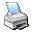Meters are used to measure current and voltage. Strictly speaking the meter actually only reads current The meter most likely encountered will be a single low range meter such as 0 - 1 ma full deflection meter of the D'Arsonval type. Other popular types will be 100 uA or other micro-ampere types. The D'Arsonval type meter works on the principle a coil of wire to which a pointer is attached is pivoted between the poles of a permanent magnet. When current flows through the coil, it sets up a magnetic field that interacts with the field of the magnet to cause the coil to turn. The meter pointer deflects in direct proportion to the current. This meter is called an ammeter.

## WWW.ELECTRONICS-TUTORIALS.COM

Authored by Ian C. Purdie VK2TIP

Text size:increasedecrease

Please visit VK2TIP's Book Shelf. My personal recommendations, thanks.

# meters�NEW! ‣ - Amazon Electronic Component Packs. Check out the Amazon Electronic Component Packs page.

### What are meters?

Meters are used to measure current and voltage. Strictly speaking the meter actually only reads current The meter most likely encountered will be a single low range meter such as 0 - 1 ma full deflection meter of the D'Arsonval type. Other popular types will be 100 uA or other micro-ampere types. The D'Arsonval type meter works on the principle a coil of wire to which a pointer is attached is pivoted between the poles of a permanent magnet. When current flows through the coil, it sets up a magnetic field that interacts with the field of the magnet to cause the coil to turn. The meter pointer deflects in direct proportion to the current. This meter is called an ammeter.Figure 1 - a typical 0 to 1 mA ammeter

### What is the accuracy of meters?

The types of meters discussed here are generally speaking, accurate to about 2% which for most practical purposes is sufficiently accurate.

### What about measuring voltage with meters?

Because of ohms law we are able to read voltage on a meter which measures current. This is called a d.c. voltmeter.

If a large resistance is placed in series with a curent reading meter as in figure 2 below the current multiplied by the resistance, I * R, will be the voltage drop across the resistance. This is called a meter multiplier and a meter calibrated in terms of the voltage drop is called a "d.c. voltmeter".Figure 2 - schematic of a meter multiplier

The real tricky part here is "sensitivity". Voltmeters are customarily graded by sensitivity expessed as ohms-per-volt. This means the full scale voltage reading multiplied by the sensitivity will give the total resistance of the voltmeter. Do NOT confuse this with the internal resistance of the meter itself (see later below).

An example of the sensitivity of a voltmeter might be a 1K ohms/volt voltmeter. Using ohms law we find the full scale deflection required is 1 mA. On the other hand, the minimum standard for a reasonable quality d.c. voltmeter is 20K ohms/volt voltmeter. This, again by ohms law, would be a 50 uA ammeter. These comments do not apply to modern digital multimeters which are something else again and will be dealt with in another series of tutorials.

The significance of the sensitivity can be amply demonstrated in the example in Figure 3 below.Figure 3 - schematic of meter sensitivity

Here we have a "true" voltage of 100 volts in a high impedance circuit. If it were measured using say a very high impedance voltmeter of say 10 megohm the meter would read around 99.9 V. On the other hand a 20K ohms/volt voltmeter would indicate around 98 V while a lowly 1K ohms/volt voltmeter would give a reading of about 80 V. Why is this so? Because in a high resistance circuit the meter tends to load down the circuit, and a voltage divider action takes place. See the topic impedance if you haven't already read it.

### How do we work out meter multipliers?

Let's consider a popular example of a 0 - 1 mA meter movement we have picked up surplus somewhere and want to incorporate it into our variable voltage power supply project. In that example we wanted a 0 - 20 V meter to monitor our output voltage. We'll say the meter internal resistance (see below later) was measured as being 200 ohms.

Rm = E / I (classic ohms law)

Where:
Rm is the total resistance of the instrument (including multiplier).
E is the desired full scale voltage reading.
I is the full scale current reading in milli-amperes.

Rm = 20 V / 0.001 = 20 V / 0.001 = 20,000 ohms

In our case we need 20,000 ohms minus the internal resistance of 200 ohms or 19,800 ohms or 19K8 net. This could be achieved by three resistors 12K, 6K8 and 1K in series, preferably the newer metal film high tolerance types. New meter faces are often drawn with graphics software, printed out and carefuly affixed to the meter. The earlier comments regarding meter sensitivity do not apply here because the power supply circuit impedance is extremely low.Figure 4 - schematic of 20 V D.C. voltmeter

### What about measuring resistance with meters?

Again because of ohms law we are able to read resistances on a meter which measures current.

 Click image to print out a printer friendly version of this page.Looking for more? Visit my site map page:

This site is hosted at Press Wizards for better value.

### Got a question on this topic?

If you are involved in electronics then consider joining our "electronics Questions and Answers" news group to ask your question there as well as sharing your thorny questions and answers. Help out your colleagues!.

This is a mutual help group with a very professional air about it. I've learn't things. It is an excellent learning resource for lurkers as well as active contributors.